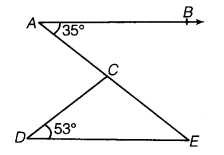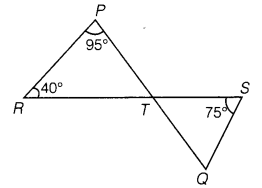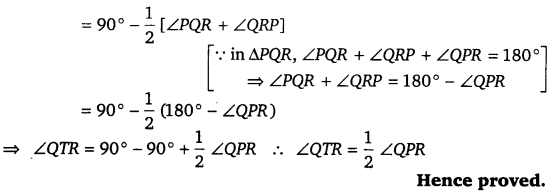# CLASS 9 MATH NCERT SOLUTION FOR CHAPTER – 6 LINES AND ANGLES EX – 6.3

## Lines and Angles

Question 1.
In the given figure, sides QP and RQ of ΔPQR are produced to points S and T respectively.

If ∠SPR = 135° and ∠PQT = 110°, find ∠PRQ.Solution:
∠RPS + ∠RPQ = 180 °
135° + ∠RPQ = 180°  [∵ ∠RPS = 135° (given)]
⇒  RPQ = 180°-135° = 45°
Now, ∠RPQ + ∠PRQ = ∠PQT  [ext. angle = sum of int. opp. angles]
⇒ 45° + ∠PRQ = 110°
⇒ ∠PRQ = 110° – 45° = 65°

Question 2.
In the given figure, ∠X= 62°,∠XYZ= 54°. If YO and ZO are the bisectors of ∠XYZ and ∠XZY respectively of ΔXYZ, find ∠OZY and ∠YOZ.

Solution:

Given, ∠X = 62° and ∠XYZ = 54°
In ΔXYZ, ∠XYZ + ∠YXZ + ∠XZY = 180° [since a sum of all the angles of a triangle is 180°]
54°+62°+∠180°⇒ 116°+∠XYZ =18O°
∠XZY=180°-116°
∴  ∠XZY = 64°
Also, given that YO and ZO are the bisectors of ∠XYZ and ∠XZY, respectively.
So, ∠OYZ =$\cfrac { 1 }{ 2 }$ ∠XYZ => ∠OYZ =$\cfrac { 1 }{ 2 }$ x 54° = 27°
and ∠OZY =$\cfrac { 1 }{ 2 }$ ∠XZY=$\cfrac { 1 }{ 2 }$ x 64°= ∠OZY=32°
Now, in ΔOYZ, ∠OYZ + ∠OZY + ∠YOZ = 180° [since, sum of all the angles of a triangle Is 180°]
27° + 32° + ∠YOZ = 180°
59°+∠YOZ = 180° ⇒ ∠YOZ = 180°—59°
∴ ∠YOZ = 121°
Hence,∠OZY = 32° and ∠YOZ = 121°

Question 3.
In figure,If AB DE, ∠BAC= 35°and ∠CDE= 53°,find ∠DCE.Solution:
We have, AB || DE
⇒  ∠AED = ∠ BAE (Alternate interior angles)
Now, ∠BAE = ∠BAC
⇒  ∠BAE=35° [ ∵ ∠BAC = 35°Given)]
∠AED = 35°
In Δ DCE,
∴ ∠DCE + ∠CED+ EDC = 180°, (∵ Sum of all angles of triangle is equal to 180°)
⇒ ∠DCE + 35° + 53° – 180°         (∵∠AED = ∠CED = 35°)
⇒  ∠DCE = 180 °-(35° + 53° ) ⇒  ∠DCE = 92°

Question 4.
In the given figure, if lines PQ and RS intersect at point T such that ∠PRT = 40°, ∠RPT = 95° and ∠TSQ = 75° then find ∠SQT.Solution:
Given, ∠PRT = 40°, ∠RPT = 95° and
∠TSQ = 75°
In Δ PRT, ∠PRT + ∠RPT + ∠PTR = 180°          …(i) [since, sum of all the angles of a triangle is 180°]
On putting ∠PRT =40° and ∠RPT = 95° in eq. (i), we get
40° + 95° + ∠PTR = 180°
⇒ 135° + ∠PTR = 180° ⇒ ∠PTR = 180° -135° ⇒ ∠PTR = 45°
Now, ∠PTR    = ∠QTS [vertically opposite angles]
∴ ∠QTS – 45°
In ∠TQS, ∠QTS + ∠TSQ + ∠TQS = 180°   … (ii)
[since, sum of all the angles of a triangle is 180°]
On putting ∠QTS = 45° and ∠TSQ = 75° in eq. (ii), we get
45° + 75° + ∠TQS = 180° ⇒120° + ∠TQS = 180°
⇒  ∠TQS    – 180°-120°
∴  ∠TQS    =60°
Hence,∠TQS    = ∠SQT = 60°

Question 5.
In the given figure, if PQ ⊥ PS, PQ || SR, ∠SQR – 28° and ∠QRT – 65° then find the values of x and y.Solution:
For Δ QSR, ∠QRT is an exterior angle
∠QRT = ∠SQR + ∠QSR [∵  exterior angle = sum of interior opposite angles]
⇒ 65° = 28° + ∠QSR [∵ ∠QRT = 65° and ∠SQR = 28°, given]
∠QSR = 65° – 28° ⇒ ∠QSR = 37°
Given PQ || SR and SQ is the transversal which intersects PQ and ST at Q and S, respectively.
∴ ∠QSR = ∠PQS  [alternate interior angles]
⇒  x = 37°
Now, in ΔPQS, ∠SPQ + ∠PQS + ∠PSQ = 180°[since, sum of all the angles of a triangle is 180°]
⇒    90° + 37° + y = 180°   [∵ PQ ⊥ PS ⇒ ∠SPQ = 90°]
⇒    127° + y= 180°⇒ y = 180° -127° = 53°
Hence, x = 37° and y= 53°.

Question 6.
In the given figure, the side QR of ΔPQR is produced to a point S. If the bisectors of ∠PQR and ∠PRS meet at point T, then prove that ∠QTR =$\cfrac { 1 }{ 2 }$ ∠QPR.Solution:
In ΔTQR, ∠QTR + ∠TQR + ∠QRT = 180[sum of all the angles of a triangle is 180°]
∠QRT = 180– [∠TQR + ∠QRT]
∠PQR + ∠QRP + ∠PRT